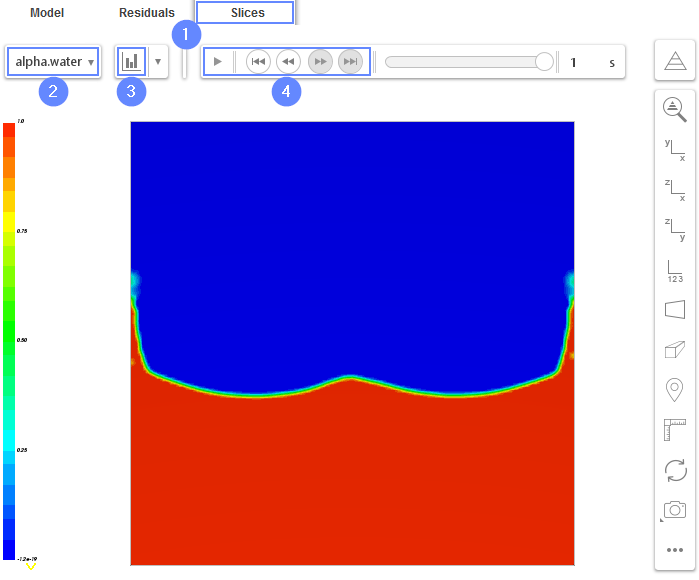Back to all tutorials

# Droplet - CFD Simulation SimFlow Tutorial

## 1. Introduction

The Droplet tutorial serves as an introduction to SimFlow. In this tutorial, we will present the simulation of free free-falling droplet into the tank (geometry created directly in SimFlow). This simulation considers a 2D analysis of a multi-phase (water + air), incompressible flow.

SimFlow is a general purpose CFD Software

## 3. Create Case

Open SimFlow and create a new case named droplet

1. Go to New panel
2. Provide name droplet
3. Click Create Case to open new project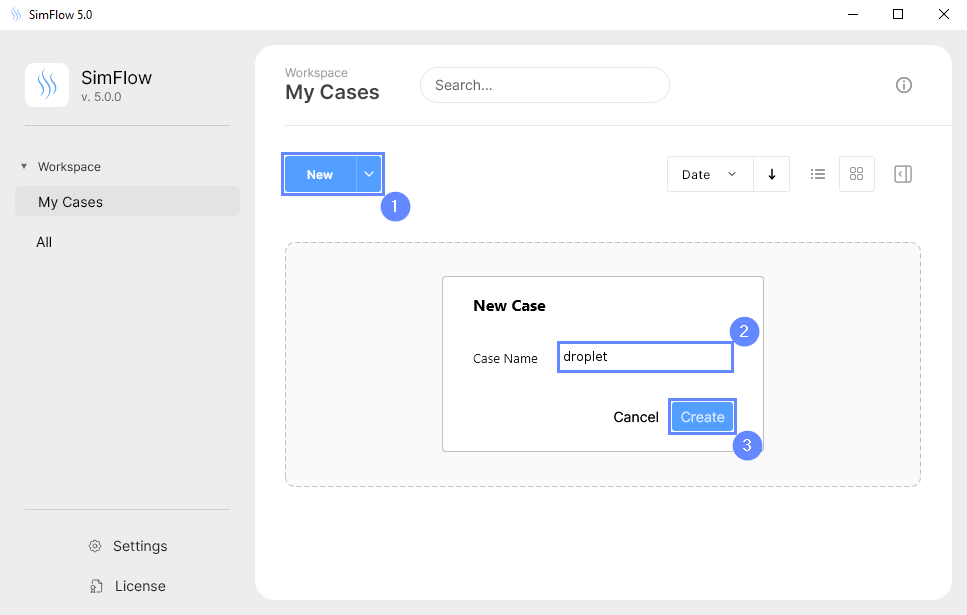## 4. Meshing parameters

We will start by creating a 2D mesh. This can be accomplished by choosing the Plate type as the background mesh.

1. Go to Hex Meshing panel
2. Go to Base tab
3. Select Plate as a Base Mesh Type
4. Define minimum and maximum extend
Min $${\sf [m]}$$00
5. Define the number of divisions
Division8080
6. Change boundary type to wall for all background mesh boundaries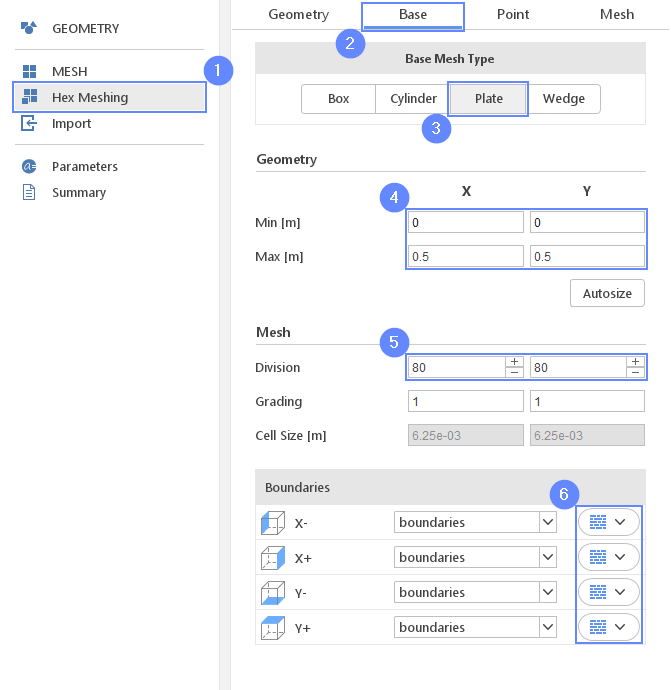## 5. Start Meshing

We will start by creating a 2D mesh. This can be accomplished by choosing the Plate type as the background mesh.

Now we are ready to create our simple mesh.

1. Go to Mesh tab
2. Press the button to start meshing process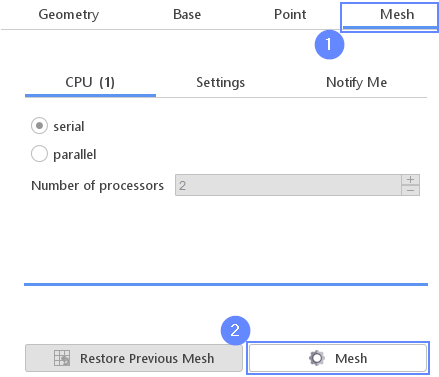## 6. Mesh

After the meshing process is finished, the mesh should appear in the graphics window.

1. Click or press CTRL+F1 to orient view plane
2. Click to zoom the geometry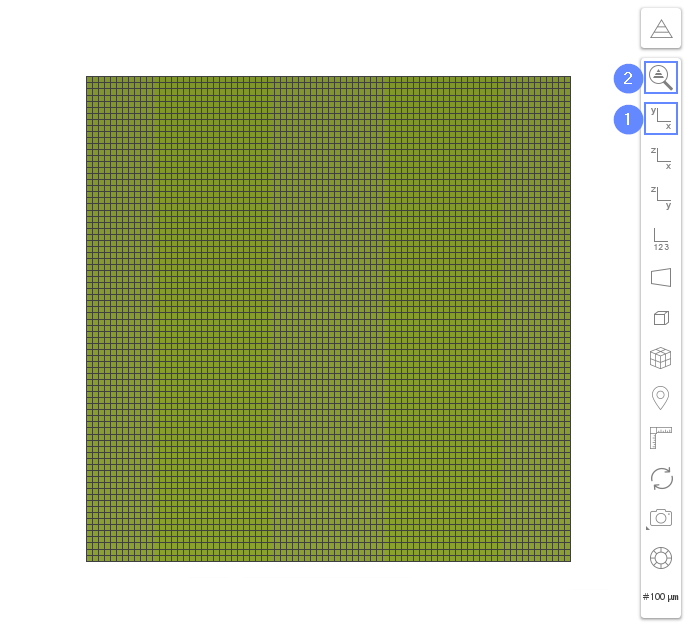## 7. Create Geometry - Droplet

To indicate the initial shape of the droplet we will use cylinder geometry.

1. Go to Geometry panel
2. Select
3. Change geometry name from cylinder_1 to droplet
4. Set the origin
Origin $${\sf [m]}$$0.250.4-0.1
5. Set the cylinder dimensions
Length $${\sf [m]}$$0.2
Radius $${\sf [m]}$$0.025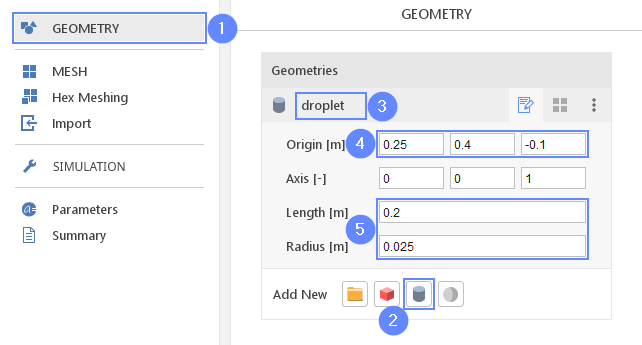## 8. Create Geometry - Water

Additionally, we would like the droplet to fall down into the tank partially filled by water. To fill the bottom part of the domain with the water we will add another geometry.

1. Add a new geometry by clicking
2. Change geometry name from box_1 to water
3. Set the origin and box dimensions
Origin $${\sf [m]}$$00-0.1
Dimensions $${\sf [m]}$$0.50.20.2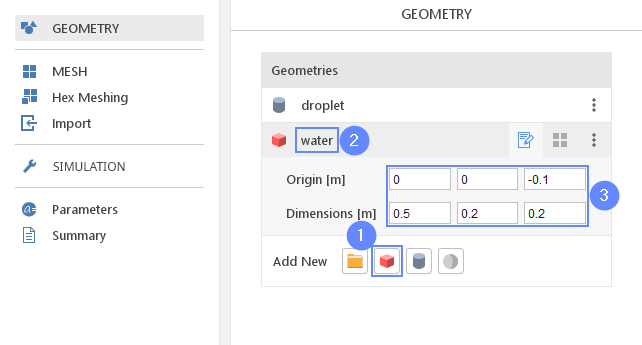## 9. Create Geometry - Water Refinement

To be able to better resolve water behavior, we will create an area with a higher mesh resolution. To do this, we will add two more box geometries.

1. Select
2. Change geometry name from box_1 to water_refinement
3. Set the origin and box dimensions
Origin $${\sf [m]}$$00-0.1
Dimensions $${\sf [m]}$$0.50.30.2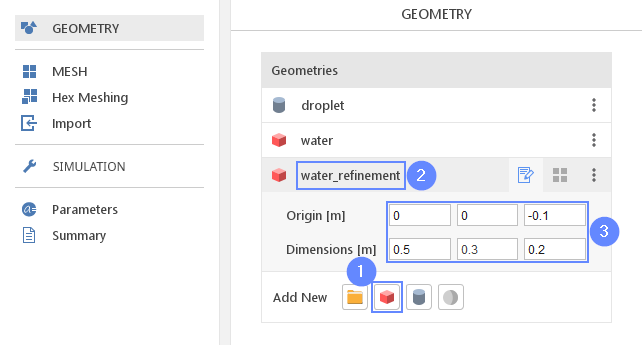## 10. Create Geometry - Droplet Refinement

The second refinement box will be located at the path of the falling droplet.

1. Select
2. Change geometry name from box_1 to droplet_refinement
3. Set the origin and box dimensions
Origin $${\sf [m]}$$0.20.3-0.1
Dimensions $${\sf [m]}$$0.10.20.2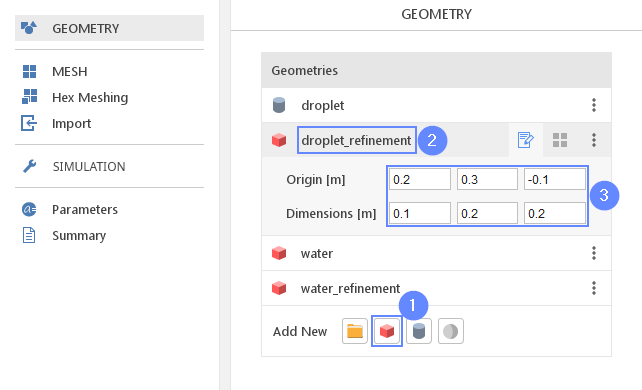## 11. Refine Mesh (I)

1. Go to Mesh panel
2. Expand the Options list next to default region
3. Select Refine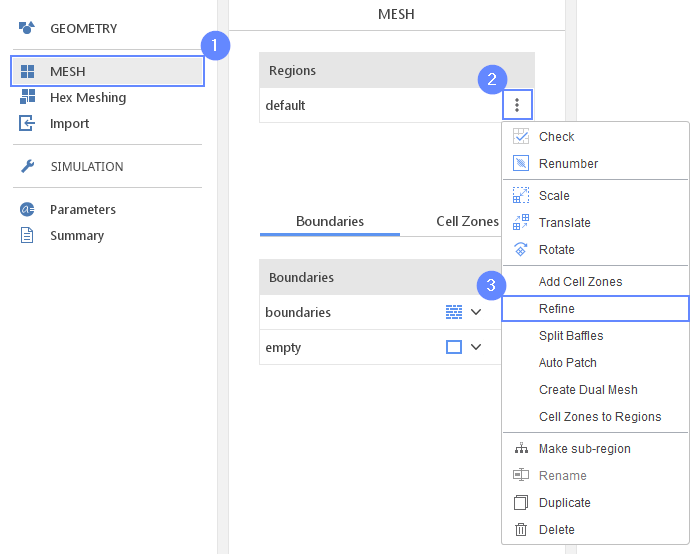## 12. Refine Mesh (II)

1. Check the refinement regions
droplet_refinement
water_refinement
2. Uncheck the axis in Refinement Directions
3. Click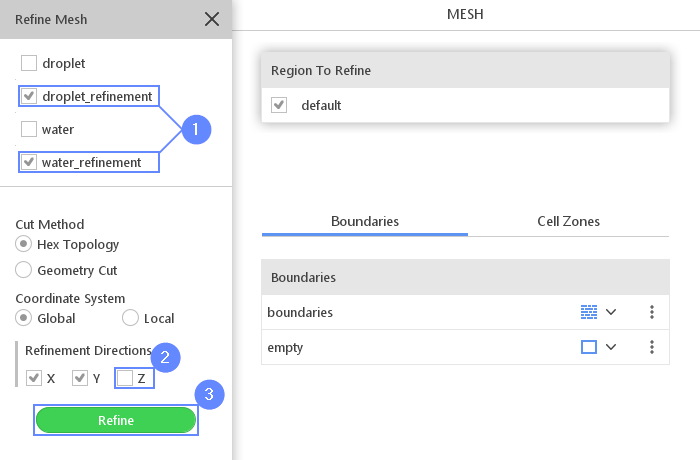## 13. Display Mesh

Check the refinement region by hiding the geometries and displaying mesh.

1. Click
2. Uncheck Geometry
 To hide the Graphics Objects panel press the Esc key.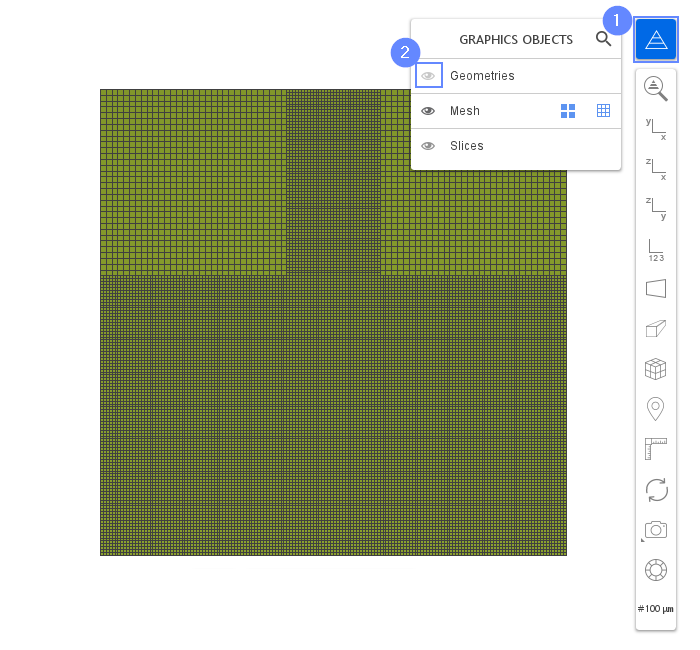## 14. Select Solver - Inter

To analyze water behavior we will use Inter (interFoam) solver. This solver designed to model two-phase flow with interface capturing capabilities.

1. Go to SETUP panel
2. Select Transient filter
3. Select Multiphase model filter
4. Pick Inter (interFoam) solver
5. Click button to confirm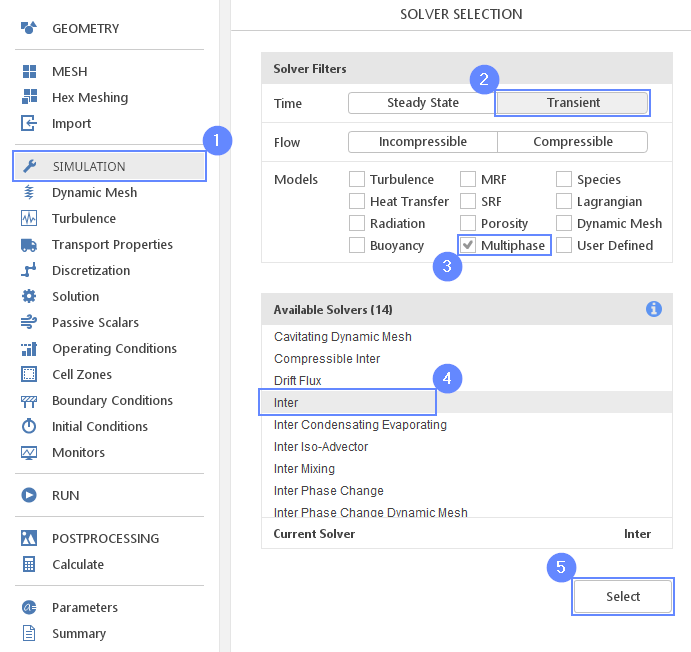## 15. Transport Properties - Water

Now we will define the transport properties for both fluids.

1. Go to Transport Properties panel
2. Change phase name from phase1 to water
3. Open
4. Pick up water from the list
5. Click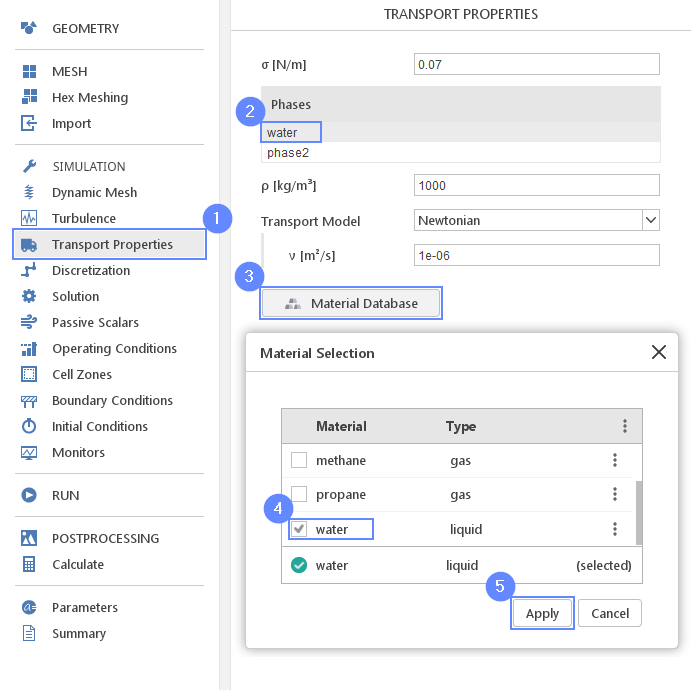## 16. Transport Properties - Air

Repeat this step for phase2 using air properties.

1. Change phase name from phase2 to air
2. Open
3. Pick up air from the list
4. Click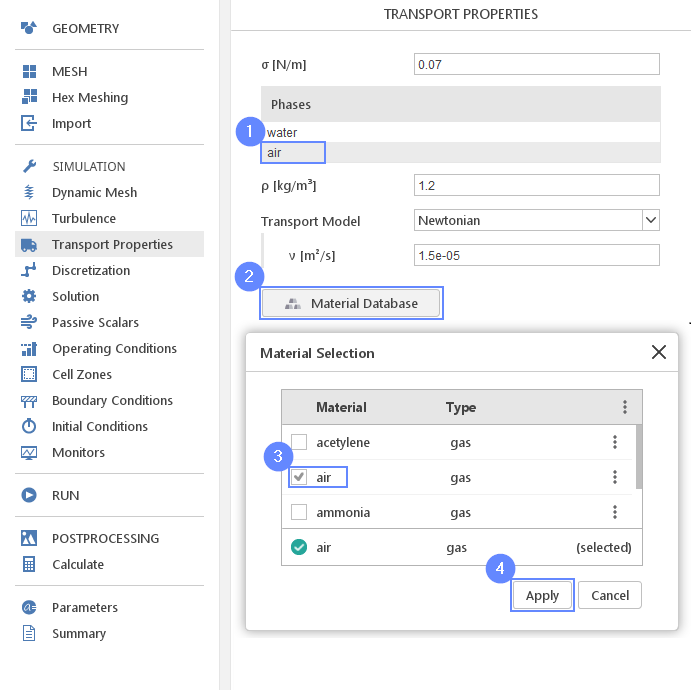## 17. Operating Conditions - Gravity

1. Go to Operating Conditions panel
2. Define gravitational acceleration along negative Y-axis
g $${\sf [m/s^2]}$$0-9.810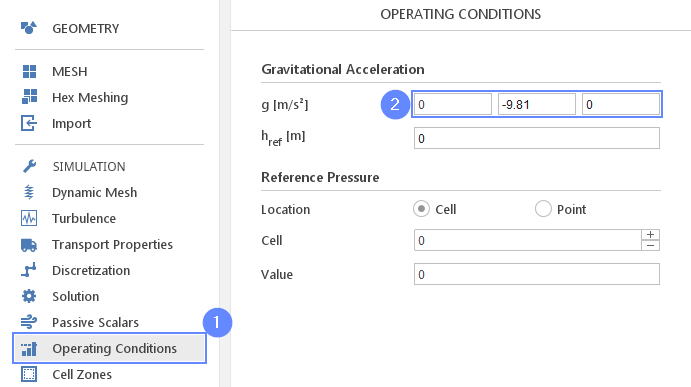## 18. Initial Conditions - Droplet

We will use the droplet geometry to select the region where water phase fraction should be initially applied.

1. Go to Initial Conditions panel
2. Switch to Patch tab
3. Enable initialization on droplet
4. Expand list
5. Select $$\alpha_{water}$$ fraction for initialization
6. Set initial value of $$\alpha_{water}$$ to 1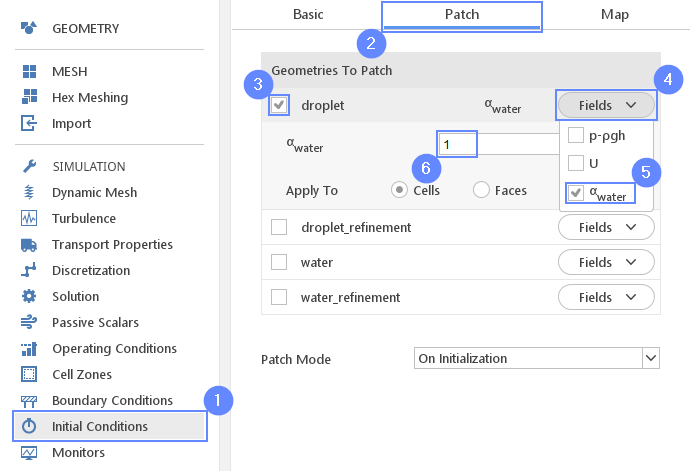## 19. Initial Conditions - Water

Repeat the step for water geometry.

1. Enable initialization on water
2. Expand list
3. Select $$\alpha_{water}$$ fraction for initialization
4. Set initial value of $$\alpha_{water}$$ to 1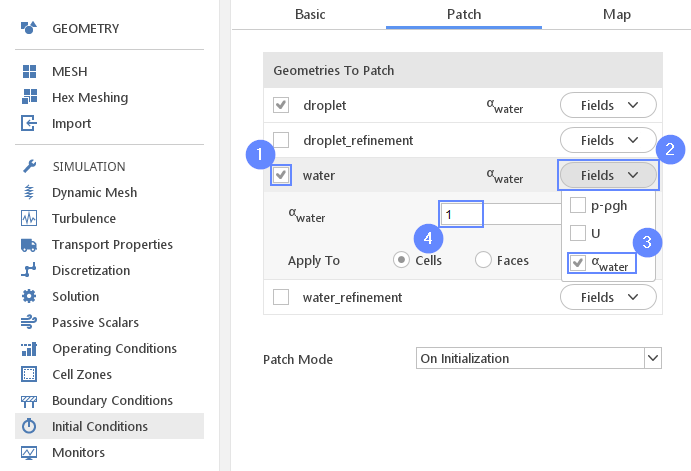## 20. Monitors - Create Slice

During calculation, we can observe intermediate results on a section plane. To add sampling data on a plane we need to define plane properties and also select variables that will be sampled. Note that runtime post-processing can only be defined before starting calculations and can not be changed later on.

1. Go to Monitors panel
2. Switch to Sampling tab
3. Select
4. Expand list
5. Check U and $$\alpha_{water}$$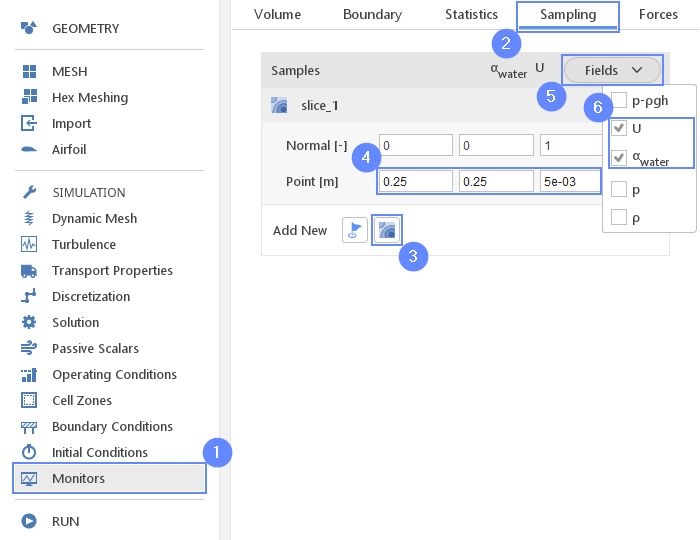## 21. Run - Time Controls

For any simulation, it is very convenient to let the solver automatically determine the proper time step value. To use this option we need to define time step constraints by providing the initial time step(adjusted by the solver during computations), maximal time step value and the Courant number. In our case, we will reduce the default Courant number for better stability and quality.

1. Go to RUN panel
2. Change Time Stepping to Automatic
3. Set initial time step, time step limit and Courant number accordingly
Initial $$\Delta t$$ $${\sf [s]}$$5e-03
Max $$\Delta t$$ $${\sf [s]}$$0.1
Max Co $${\sf [-]}$$0.5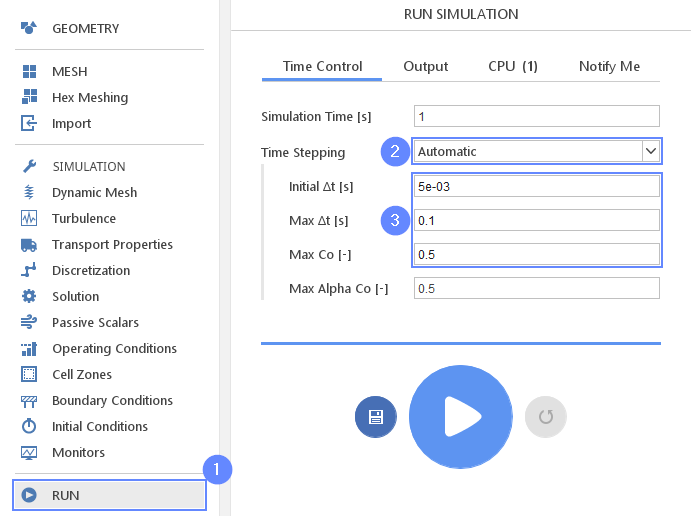## 22. Run - Output

It is very important to control when results should be stored on the hard drive. This is especially important for the transient simulations where users are interested in the whole flow history saved as a collection of the snapshots.

1. Switch to Output tab
2. Set Write Control Interval [s] to 0.02
(solver will store results on the hard drive every 0.02 second of the simulation)
3. Click button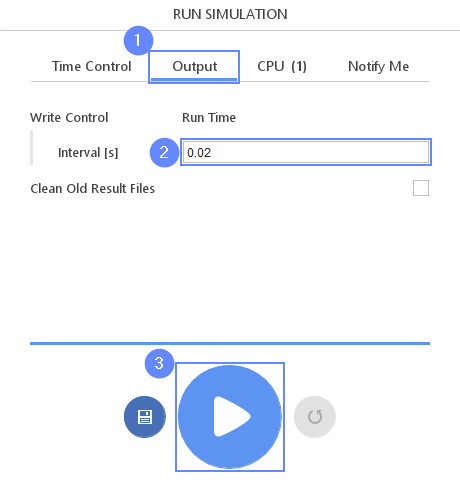## 23. Results

When calculations will begin SimFlow automatically will switch view to the Residuals tab, where we can observe the convergence of our simulation. This is very handy for steady-state simulations when we try reaching low residuals levels. In case of transient simulation, we would rather like to see how our flow develops as simulation time progress.

1. Switch to Slices tab
2. Choose field
3. Click
4. Play with an animation buttons to track the results of analysis